# Multicylinder Pneumatic Circuits & Electropneumatics - MCQs with Answers

## Multicylinder Pneumatic Circuits & Electropneumatics – MCQs with Answers

1. Which of the following is the correct displacement diagram for a single acting cylinder, where 0 and 1 on the left hand side are initial and final positions of piston rod respectively and a, b, c are stages of extension and retraction?

a.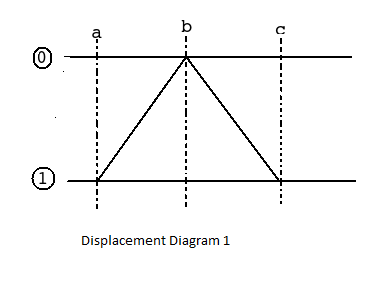b.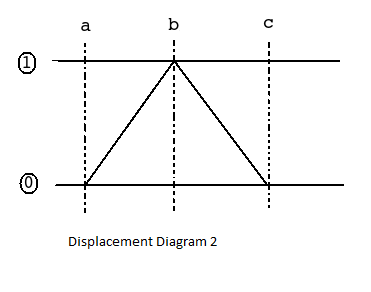c.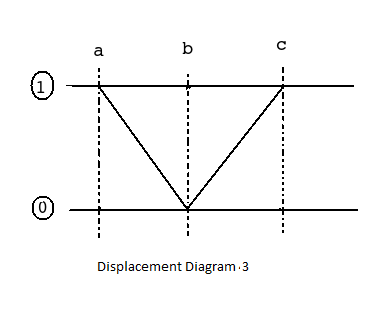d.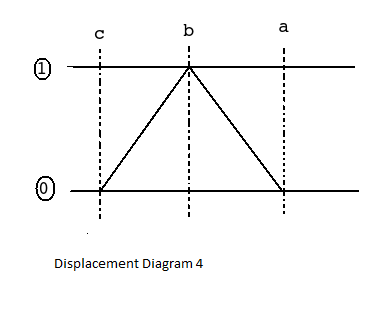2. Overlapping of signals in pneumatic systems can be avoided by using

a. rolling lever valve
b. idle roller lever valve
c. both a and b
d. none of the above

ANSWER: c. both a and b

3. What is the notation used for the sequence of operations mentioned below?

1. Cylinder A undergoes forward stroke
2. Cylinder B undergoes forward stroke
3. Cylinder A undergoes backward stroke
4. Cylinder B undergoes backward stroke

a. A B A+ B+
b. A+ B A+ B
c. A+ B+ A B
d. A+ B
A+ B

4. Which of the following statements is true for cascade method which is used to draw a pneumatic circuit?

a. signal processing valves are connected in parallel
b. when the number of signal processing valves are greater than 4, the signals are strong
c. cascade method does not consider the cost factor
d. all the above

5. What is the part, shown in below diagram of 3/2 valve, called?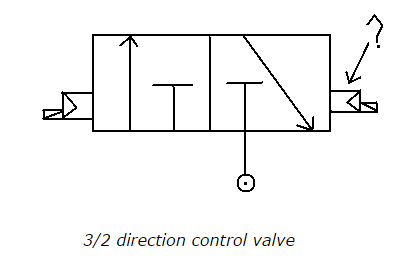a. manually operated valve
b. pilot operated valve
c. pressure electric converter
d. none of the above

6. How is proximity switch differentiated from limit switch?

a. proximity switch is activated when moving parts have physical contact with it
b. proximity switch is activated when non-moving parts have physical contact
c. proximity switch is activated when moving parts are close to it
d. none of the above

ANSWER: c. proximity switch is activated when moving parts are close to it

7. Which of the following statements is true?

a. electromagnetic relays have high reliability at more cost

b. electromagnetic relays use low current and voltage, to have open or close contact in high voltage and current circuit

c. air pressure passed to pressure electric converter opens a contact which energizes a circuit for the flow of electric contact

d. all the above

ANSWER: b. electromagnetic relays use low current and voltage, to have open or close contact in high voltage and current circuit

8. In which circuits, relay of low voltage and low current is used to make open or close contact?

a. high voltage and high current circuit
b. low voltage and low current circuit
c. high voltage and low current circuit
d. low voltage and low current circuit

ANSWER: a. high voltage and high current circuit

9. In electropneumatic circuits,

a. spool is shifted by signal air
b. spool is shifted by control air
c. spool is shifted by electromotive force
d. all the above

ANSWER: c. spool is shifted by electromotive force

10. Why are electromechanical relays more popular than solid state relays?

a. they are reliable
b. less costly
c. both a and b
d. none of the above

ANSWER: c. both a and b

11. How is strong magnetic field in a solenoid achieved ?

a. strong magnetic field in a solenoid is achieved, if coil acts as conductor
b. coil is surrounded by a iron frame
c. iron core is placed at the centre of the coil
d. all the above

12. What is the DC range of of solenoids in pneumatic systems?

a. 12 V and 24 V
b. 110 V and 220 V
c. both a and b
d. none of the above